# 夯实基础中篇-图解作用域链和闭包

1. 什么是作用域链
2. 什么是词法作用域
3. 什么是闭包
4. 闭包的实际使用案例

## 什么是作用域链

``````    function test() {
console.log(name)
}
function test1() {
const name = 'test1的name'
test()
}
const name = 'global的name'
test1()
``````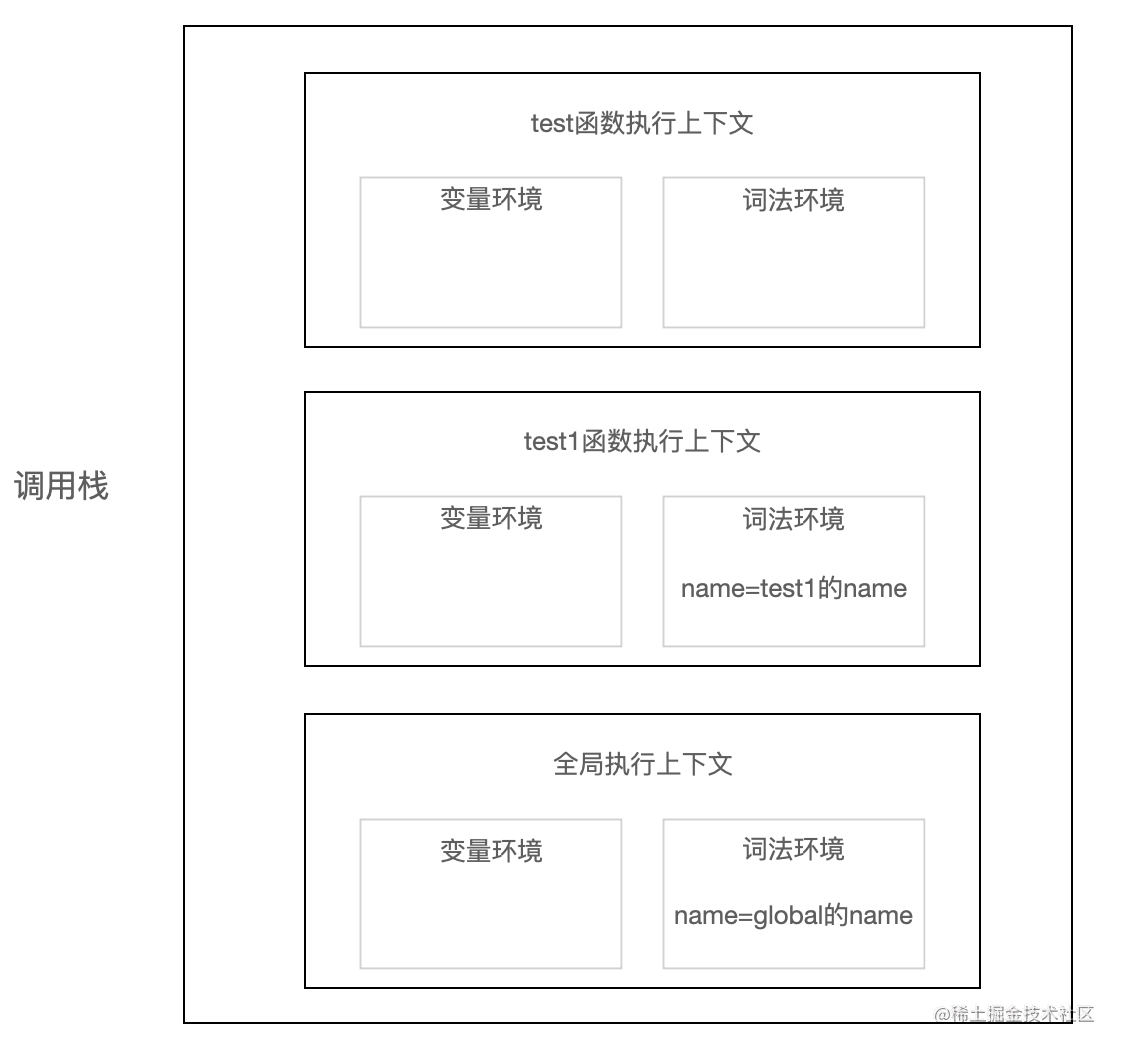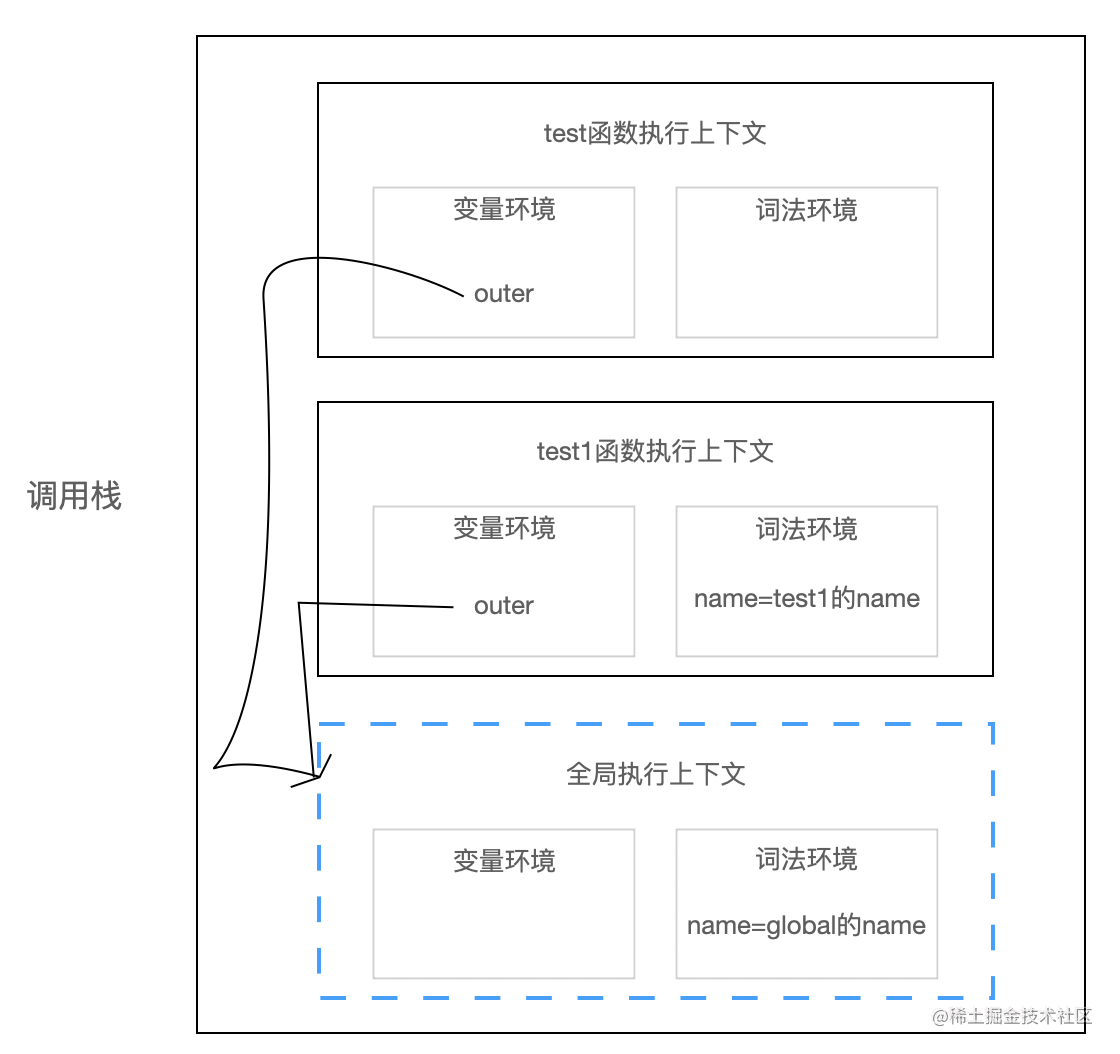## 什么是词法作用域

``````    const count = 0
function test() {
const count = 1

function test1() {
const count = 2

function test2() {
const count = 3
}
}
}
``````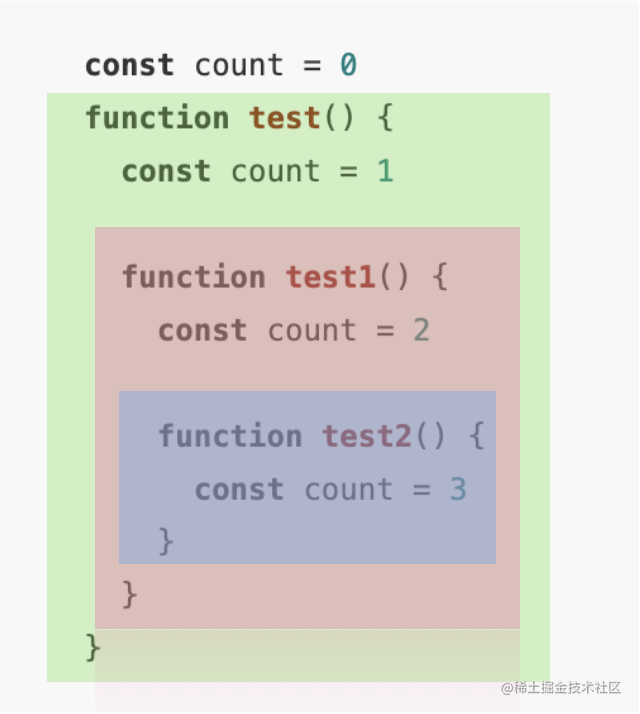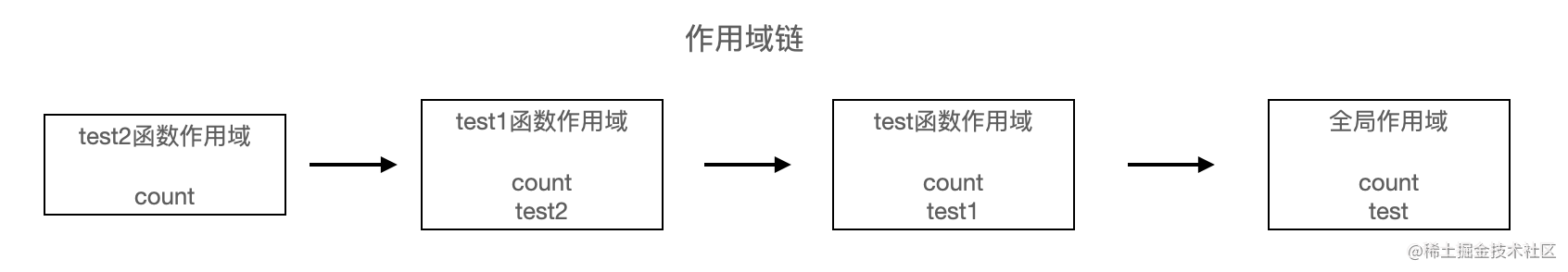## 什么是闭包

``````    var globalVariable = 1
const scriptVariable = 2

function test() {
let name = 'Jaychou'

return {
getName() {
const count = 1
return name
},
setName(newValue) {
name = newValue
}
}
}

const testFun = test()
console.log(testFun.getName()) // Jaychou
testFun.setName('小明')
console.log(testFun.getName()) // 小明
``````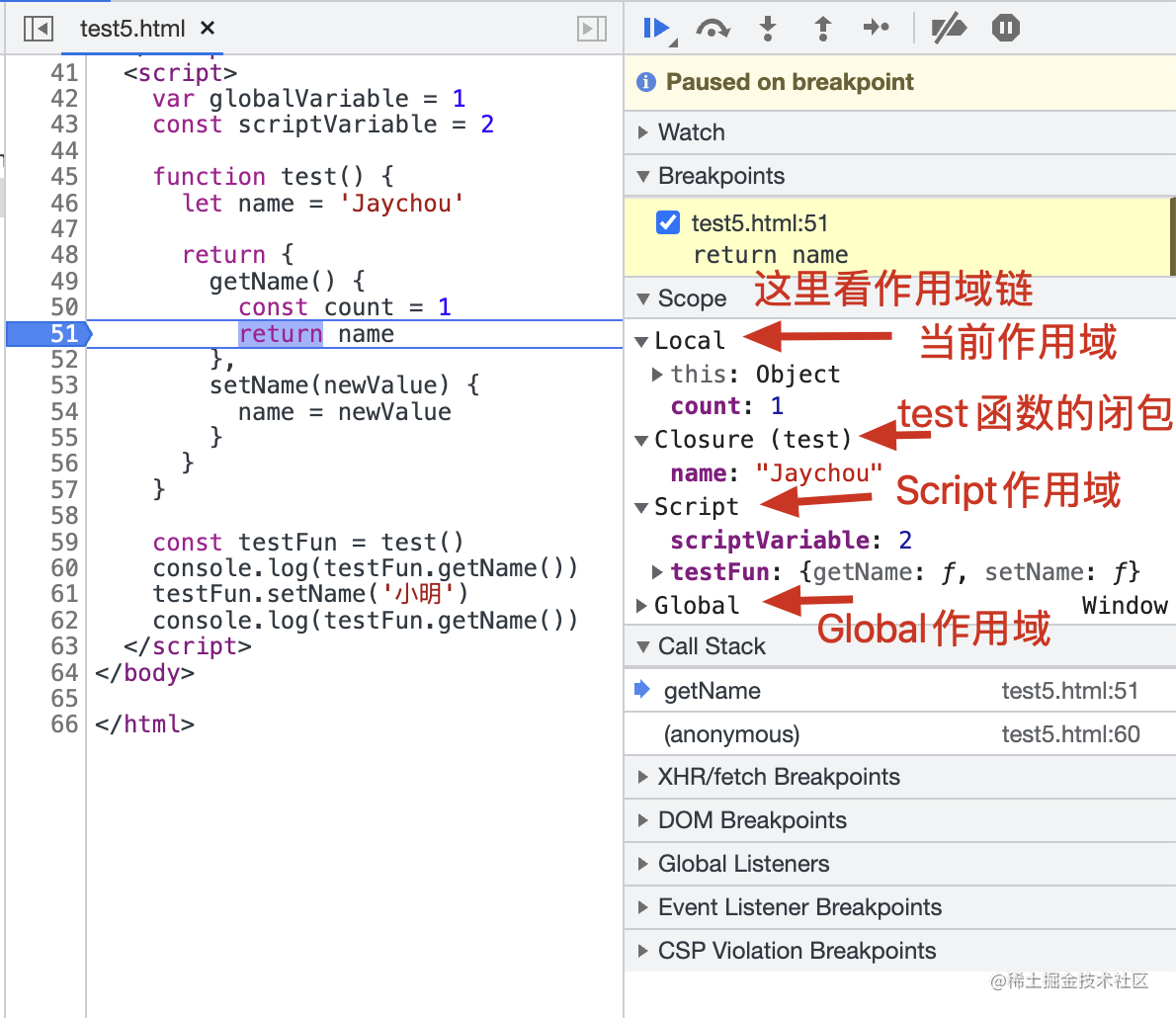1. 为什么叫 test 函数的闭包？因为当`const testFun = test()`的 test 函数执行完之后，test 的函数执行上下文已经被销毁了，但它返回的`{ getName(){}, setName(){} }`对象被 testFun 引用着，而 getName 和 setName 引用着 test 函数内定义的 name 变量，所以这些被引用的变量依然需要被保存在内存中，而这些变量的集合称为闭包 Closure；
2. 目前闭包内的 name 变量就只能通过 getName 和 setName 去访问和设置，而这也是闭包的作用之一：封装私有变量；
3. 刚才说的 Script Scope 中保存着 scriptVariable 变量，globalVariable 变量是 var 声明的，所以在 Global Scope（Window）中。

``````    const globalCount = 0

function test() {
const count = 0
return test1

function test1() {
const count1 = 1
return test2

function test2() {
const count2 = 2
console.log('test2', globalCount + count + count1 + count2)
}
}
}
test()()()
``````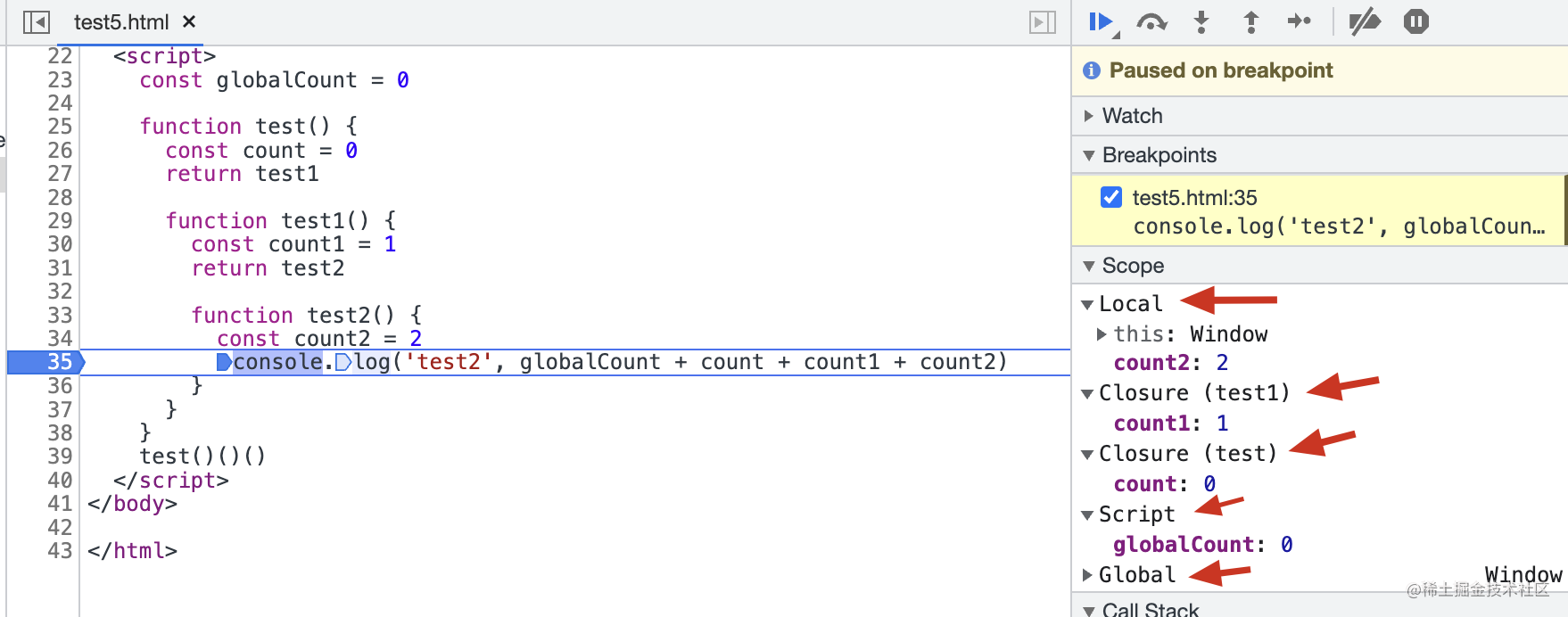## 闭包的实际使用案例

### 封装单例

``````    const Single = (function () {
let instance = null

return function () {
if (!instance) {
instance = {
name: 'jaychou',
age: 40
}
}
return instance
}
})()
const obj1 = new Single()
const obj2 = new Single()
console.log(obj1 === obj2) // true
``````

### 防抖和节流

``````    function debounce(fn, delay) {
let timer = null;

return function () {
let context = this;
let args = arguments;

timer && clearTimeout(timer);

timer = setTimeout(function () {
fn.apply(context, args);
}, delay);
}
}
``````

``````    function throttle(fn, interval) {
let last = 0;

return function () {
let now = +new Date()
if (now - last >= interval) {
fn.apply(this, arguments);
last = now;
}
}
}
``````